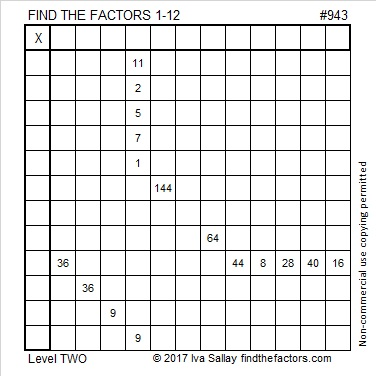# 943 and Level 2

Level 2 puzzles aren’t very tricky, but maybe this one is a little bit. Can you write the factors from 1 to 12 in both the first column and the top row so that this puzzle functions as a multiplication table?Print the puzzles or type the solution in this excel file: 12 factors 942-950

Now I’ll write something about the number 943:

943 is the hypotenuse of a Pythagorean triple:
207-920-943 which is 23 times (9-40-41)

It is also a leg in two primitive Pythagorean triples:
576-943-1105, calculated from 2(32)(9), 32² – 9², 32² + 9²
943-444624-444625, calculated from 472² – 471², 2(472)(471), 472² + 471²

• 943 is a composite number.
• Prime factorization: 943 = 23 × 41
• The exponents in the prime factorization are 1 and 1. Adding one to each and multiplying we get (1 + 1)(1 + 1) = 2 × 2 = 4. Therefore 943 has exactly 4 factors.
• Factors of 943: 1, 23, 41, 943
• Factor pairs: 943 = 1 × 943 or 23 × 41
• 943 has no square factors that allow its square root to be simplified. √943 ≈ 30.708305This site uses Akismet to reduce spam. Learn how your comment data is processed.